## Mathematical Functions

Most of the new features of the SB-6668 counter are related to mathematical functions. Because these functions both apply to manual and automated control it's best that I explain the new mathematical functions first. The purpose of the mathematical functions is to modify the measured value in such a way that you can read the wanted property directly from the display. There are a few situations where these mathematical functions are very useful.

Sometimes you may be interested in an RPM value of a rotating object rather than in a frequency. Remember that frequency is measured with a reference to one second, while RPM has a 60 second reference. So you'll have to multiply the measured frequency by 60 to get the RPM value.
But suppose that the rotating object generates 4 pulses per revolution. Now you'll have to divide the measured value by 4 again to obtain the real value. You can use the "scaling" function of the counter to make these kind of calculations automatically. The scaling factor can be set to multiply the measured frequency by 60/4=15, and the counter will display the RPM value instead of the actual measured frequency.

Another good example is when you want to know the frequency a receiver is tuned to. This can usually be determined by measuring the Local Oscillator's frequency. You may measure the Local Oscillator with a pick-up coil, or you could measure its frequency behind a pre-scaler to avoid loading the oscillator. Such a pre-scaler usually has a division ratio of 16, 64, 256 or 512. Naturally other division ratios are also quite possible.
If you do measure the frequency behind such a pre-scaler you'll have to multiply the measured value by the same factor used in the pre-scaler to get the actual Local Oscillator frequency back.
But knowing the Local Oscillator isn't enough, because there is an offset which is equal to plus or minus the IF frequency. Common offsets are 450kHz, 10.7MHz and 38.9MHz, but again many others may exist.
The offset function of the SB-6668 can now be used to add or subtract this offset from the measured value, resulting in the exact frequency the tuner is tuned to.

I have added one more feature that may be considered a mathematical function, which is the SPEED function. This function allows you to measure the tape speed of e.g. a cassette deck or tape recorder. You need a standard tape, with a known constant tone to measure the tape speed. Two main standards exist: CCIR which uses a constant tone of 3000Hz, and DIN which uses a constant tone of 3150Hz.
Measuring the frequency from a standard tape is nothing special for a frequency counter, so what is so special about the SPEED function then? A 4 digit resolution is more dan enough for this purpose The remaining 3 digits in the display can be used for other purposes. We can use these free digits to display the deviation from the nominal frequency, making it easier to tune the speed.
Below you see some example displays with different values, using a CCIR standard tape: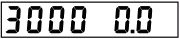Frequency = 3000 HzDeviation = 0%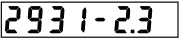Frequency = 2931 HzDeviation = -2.3%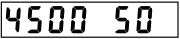Frequency = 4500 HzDeviation = +50%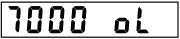Frequency = 7000 HzDeviation > 99%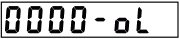Frequency = 0 HzDeviation > -99%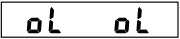Frequency > 9999 HzDeviation > 99%

The speed function can also be combined with the offset and scaling functions. Suppose you are tuning the high speed copy function of a double cassette deck, which generates a frequency of 6000Hz. You can set the Scaling function to divide the measured frequency by 2 and use that value to display the percentage properly.

Please Note: The tone produced by a cassette deck is never free from unwanted components, like hum, rumble and large amplitude deviations. Therefore it will be hard to obtain a stable trigger point for the counter. You may have to use a simple tone filter to get rid of most of the unwanted frequencies to improve the stability of the triggering.

## Scaling And Offset

The two main mathematical functions are Scaling and Offset. The measured frequency can be multiplied with the Scaling factor, or the measured frequency can be divided by the Scaling factor. You decide whether to multiply or divide when you choose the Scaling factor.
The Offset value can be added to or subtracted from the measured frequency, also on your request.

It is very important in which order the calculations take place. Sometimes you need to calculate the Scaling factor before the Offset, while in other circumstances it must be the other way around.
The order in which you activate the Scaling and Offset functions determines the order in which the calculations will take place. If you activate the Scaling function before the Offset function, then the counter will first multiply or divide the Scaling factor, and after that it will add or subtract the Offset value. But if you activate the Offset function before the Scaling function the calculation order is swapped. Needless to say that you should take great care in which order you activate both functions.
Once the calculation order is determined, it will remain true until at least one of the functions is cancelled. So you may change the actual Scaling factor and Offset values as often as you like, without affecting the calculation order. If you deactivate one of the functions (by selecting the value "NONE"), the remaining function will get the highest priority should the cancelled function be activated again.
If you're unsure of the calculation order you can always execute a RESET to clear all mathematical functions at once to start all over again.

The SPEED function can be combined with the Scaling and Offset functions. Scaling and Offset functions are always calculated before the SPEED is calculated.

One could expect the counter to display the offset value if no signal is applied to the counter, either positive or negative. To avoid confusion I decided not to allow that, and the display shows 0000000 if no signal is applied to the input, even if an Offset value is activated.

## Counter Resolution

The native resolution of the PM-6668 is 7 digits if the selected gate time is 1 second. Values above 2000000 will be displayed with only 6 digits if the selected gate time is 0.2 seconds (RATE=FAST). It is fairly useless to display more digits, for they will not be accurate due to the way the results are calculated and the fact that the reference oscillator can produce only a 7 digit value in 1 second gate time.
Please note that 2000000 is the mantissa only, without the exponent. So it may be 20.00000Hz, or 200.0000kHz, or whatever other absolute value.

The counter will remember the original resolution of a value, and will not change it after execution of the Scaling and Offset functions.

The counter uses a +10% hysteresis when switching from 7 to 6 digit resolution to avoid a nervous display. This means that the counter will not switch to 6 digit resolution until the value exceeds 21999999 counts. It will switch back to 7 digit resolution again when the reading drops below 2000000 counts.
Remember that this resolution switching only applies if the Fast measuring rate is selected. The resolution is always 7 digits when the Slow measuring rate is selected, regardless of the measured value.

Especially the Scaling function can change the displayed value dramatically. Therefore the display range is no longer limited to the actual bandwidth of the counter, which normally runs from 10Hz to 1GHz. In order to display smaller and larger values the counter will shift its decimal point to the appropriate location. We don't have additional annunciators to indicate Tera Herz for instance. So the minimum value that can be displayed is 0.000001Hz, and the maximum value is 9999999GHz. Larger values will result in "OL" for overload to be displayed
This limitation doesn't apply to the SB-Bus values because there it is a lot easier to show any reasonable exponent value.